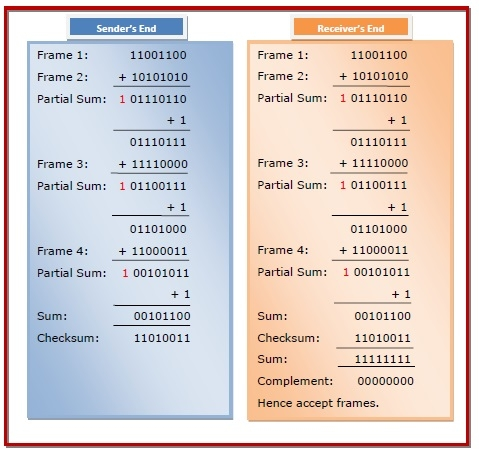# Error-Detecting Codes - Checksums

## Errors and Error Detection

When bits are transmitted over the computer network, they are subject to get corrupted due to interference and network problems. The corrupted bits leads to spurious data being received by the receiver and are called errors.

Error detection techniques are responsible for checking whether any error has occurred or not in the frame that has been transmitted via network. It does not take into account the number of error bits and the type of error.

For error detection, the sender needs to send some additional bits along with the data bits. The receiver performs necessary checks based upon the additional redundant bits. If it finds that the data is free from errors, it removes the redundant bits before passing the message to the upper layers.

There are three main techniques for detecting errors in frames: Parity Check, Checksum and Cyclic Redundancy Check (CRC).

## Checksums

This is a block code method where a checksum is created based on the data values in the data blocks to be transmitted using some algorithm and appended to the data. When the receiver gets this data, a new checksum is calculated and compared with the existing checksum. A non-match indicates an error.

## Error Detection by Checksums

For error detection by checksums, data is divided into fixed sized frames or segments.

• Sender's End − The sender adds the segments using 1’s complement arithmetic to get the sum. It then complements the sum to get the checksum and sends it along with the data frames.

• Receiver's End − The receiver adds the incoming segments along with the checksum using 1’s complement arithmetic to get the sum and then complements it.

If the result is zero, the received frames are accepted; otherwise they are discarded.

## Example

Suppose that the sender wants to send 4 frames each of 8 bits, where the frames are 11001100, 10101010, 11110000 and 11000011.

The sender adds the bits using 1s complement arithmetic. While adding two numbers using 1s complement arithmetic, if there is a carry over, it is added to the sum.

After adding all the 4 frames, the sender complements the sum to get the checksum, 11010011, and sends it along with the data frames.

The receiver performs 1s complement arithmetic sum of all the frames including the checksum. The result is complemented and found to be 0. Hence, the receiver assumes that no error has occurred.Updated on: 13-Sep-2023

24K+ Views